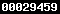## Pi day (3/14)

Today is the Pi day, March 14th, i.e. 3/14 in the American notation. As a Mathematician and Statistician, it is difficult for me not to marvel about the ways in which special numbers likeappear in many different, apparently unrelated places in Maths, Stats and real life.is of course a ratio of a circle circumference and diameter.

The Wolfram’s Mathworld lists many different formulas, either infinite series or products, that produce the number. I found some of these formulas amazing and some frankly bizzare. There is a part of my scientific background that is deeply rooted in Mathematics (thanks to my lecturers at the Jagiellonian University where I did my undergraduate and PhD studies) that causes me to stop and think, why:for example?

To me, there is an intrinsic beauty in such mathematical formulas, similar to art, e.g. Horse riders by Escher, or nature:There is another, much more chaotic, side of– the apparent unpredictability of its digits.is supposedly a normal number in that if in its sequence of digits, all digits and all subsequences of digits are equally represented. Although it has not been proven thatis indeed normal, this property seems to be well supported. In other words, if I tell you that the fifth digit is 5, each of the 10 digits (0, 1, 2, …, 9) can come next and so I cannot really conclude that sixth digit is more likely to be 9 (3.14159…).

If we consider a ‘random’ walk governed by digits of, i.e. at each time step a particle moving either to the left or to the right by how far the current digit is from the mean of 5:

• if the digit is 3, move by 2 to the left;
• if the digit is 1, move by 4 to the left;
• if the digit is 4, move by 1 to the left;
• if the digit is 5, move by 1 to the right;
• if the digit is 9, move by 4 to the right;

we get the following graph:which looks random!

I find it really amazing that there is so much to a humble number\piand that it shows so much simplicity and at the same time so much complication, so much structure, and at the same time so much randomness and unpredictability.

If you want to see more information on the randomness aspects of\pi, please see the slides from my public lecture given at the University of Stirling in 2013. You can find it at SlideShare.

I better stop now, as it is time for lunch:Broccoli picture by Jon Sullivan - http://pdphoto.org/PictureDetail.php?mat=pdef&pg=8232, Public Domain, https://commons.wikimedia.org/w/index.php?curid=95997

Posted on Categories English